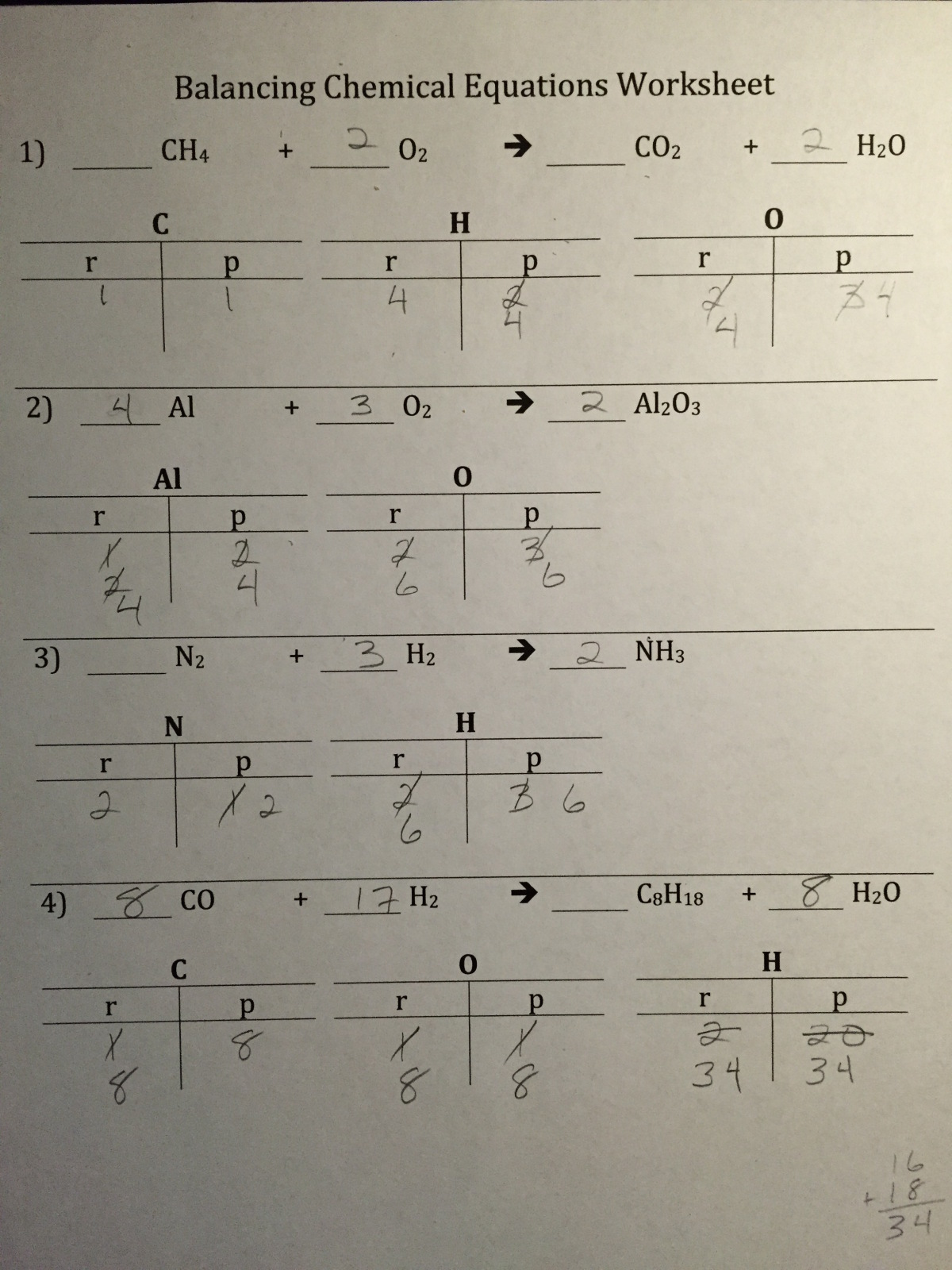# Balancing Chemical Equations

23 teachers like this lesson
Print Lesson

## Objective

Students will be able to apply the Law of Conservation of Mass to balancing chemical equations.

#### Big Idea

Atom are never created or destroyed in chemical reactions, they are simply rearranged.

## NGSS Background

This lesson is based on California's Middle School Integrated Model of NGSS.

NGSS Performance Expectation: MS-PS1-5 - Develop and use a model to describe how the total number of atoms does not change in a chemical reaction and thus mass is conserved.

Disciplinary Core Idea: PS1.B: Chemical Reactions – The total number of each type of atom is conserved, and thus the mass does not change.

Science and Engineering Practice 2: Developing and Using Models

Crosscutting Concepts: Energy and Matter

Chemical reactions are mysterious things that happen at a molecular level that may be hard for students to grasp. Atoms must abide by the Law of Conservation of Mass, which states that atoms cannot be created or destroyed in chemical reactions, atoms are simply rearranged (PS1.B).

Balancing chemical reactions is a skill that improved with repetition. Periodic Table Puzzle pieces are used to show students that atoms are rearranged in chemical reactions (MS-PS1-5, SP2). The Law of Conservation of Mass applies to matter and explains a fundamental concept in chemistry, but can also be applied to the conservation of energy (CCC Energy and Matter - Matter is conserved because atoms are conserved in physical and chemical processes).

## Teacher Set-up

50 minutes

As similiar to Periodic Table Puzzle construction glue Balancing Equations using Periodic Table Puzzle Pieces onto the back side of the puzzle pieces. I assign this repetitive job to my student teaching assistants.## Student Activity

30 minutes

Balancing chemical equations is a math-like skill that can be achieved through repitious practice. Start out with simple chemical equation and gradually build to greater difficulty. Often students need to see the process many times until an 'ah-ha' moment occurs.

I first teach the concept of balancing chemical equations (see video below). Project the Balancing Chemical Equations Board Examples and use a white board marker to walk your students through the process. Use Balancing Chemical Equations Board Examples - ANSWERS to check that the coefficients are correct.

As I am teaching, my students are writing down the examples with me.We then work together (same problems) using Balancing Chemical Equations Puzzle Pieces. As the Powerpoint is projected the students follow along with their own puzzle pieces (back side of Periodic Table Puzzle -- see that lesson).

For homework I assign Balancing Equations Worksheet and review Balancing Equations Worksheet - ANSWERS the next day.